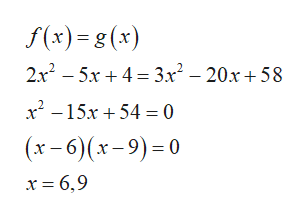# Find the area, in square units, bounded above by f(x)=2x2−5x+4 and below by g(x)=3x2−20x+58.

Question
1 views

Find the area, in square units, bounded above by f(x)=2x2−5x+4 and below by g(x)=3x2−20x+58.

check_circle

Step 1

To determine the area between the curves, first find the ex...help_outlineImage Transcriptionclosef(x) = g (x) 2x – 5x + 4 = 3x² – 20x + 58 x -15x + 54 = 0 (x - 6)(x-9)=0 x = 6,9 fullscreen

### Want to see the full answer?

See Solution

#### Want to see this answer and more?

Solutions are written by subject experts who are available 24/7. Questions are typically answered within 1 hour.*

See Solution
*Response times may vary by subject and question.
Tagged in

### Other